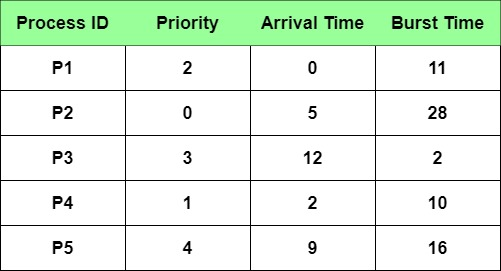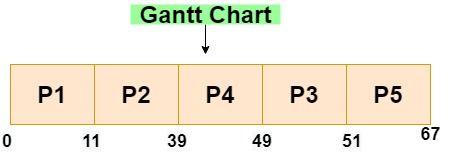New update is available. Click here to update.

# Non-Preemptive Priority Based Scheduling

## Introduction

We know that various scheduling algorithms are used to assign resources to perform different tasks. One of these CPU algorithms is Priority based scheduling.

As the name itself suggests, it is related to the priority of the task to be performed.

In a nutshell, a priority is associated with each process, and the CPU is allocated to the process with the highest priority.

Where is it used?

It is used in operating systems to perform batch processes.

Now, a natural question comes to mind - On what factors the priorities are based on?

See, the priority of a task can be decided either externally or internally.

Some of the internal factors can be -

• time limits
• memory requirements of the process
• the ratio of average I/O burst to average CPU burst.

Whereas the external factors can be -

• amount of funds allotted for computer use
• the sponsoring department of the work, etc.

If two or more tasks have equal priority, they are executed on FCFS(first come, first serve) basis, i.e., the process that arrived first among them will be given CPU first.

How are the priorities represented?

These are generally depicted by some fixed range of numbers, like 0 to 7 or 0 to 4095. Depending on the systems, the high priority can be represented by either low numbers or by high numbers.

Note: Throughout this article, we will be considering low numbers as a high priority.

We know about the SJF(shortest job first) scheduling algorithm. An interesting point here, it is also a type of priority based scheduling algorithm in which the priority of a process is inversely proportional to the next CPU burst. It means that the process with the next smallest CPU burst will have higher priority and will be assigned CPU first and vice versa.

If you are not aware of the SJF algorithm, you can read about it here.

## Types of Priority Based Scheduling

1. Preemptive Scheduling
2. Non-Preemptive Scheduling

### Preemptive Scheduling

It is a priority scheduling algorithm in which the CPU is preempted when a new process arrives i.e. it will start executing the new process if the newly arrived process is of higher priority than the currently running process. To read more about preemptive scheduling you may refer to this.

### Non-Preemptive Scheduling

In this algorithm, if a new process of higher priority than the currently running process arrives, then the currently executing process is not disturbed. Rather, the newly arrived process is put at the head of the ready queue, i.e. according to its priority in the queue. And when the execution of the currently running process is complete, the newly arrived process will be given the CPU.

### Example Of Non-Preemptive Priority Scheduling

Problem Statement - Schedule the following processes according to the Non-Preemptive Priority Scheduling algorithm

Note: In this article, we will be considering, the lesser the numeric value of priority, the higher the priority of the process. Eg- A process having priority=1 will be considered to have higher priority than a process having priority=2.

This convention may vary at different places but it will always be mentioned which convention is being followed.Steps -

• First, the process with the lowest arrival time is scheduled. If two or more of them have the lowest arrival time, they are scheduled according to their priority.
• Then, other processes will be scheduled according to their arrival time and priority. If two processes have the same priority they are sorted according to their process number.
• When a process is still in execution and few processes arrive, then they are scheduled in the ready queue according to their priority.
• This continues till all processes are scheduled.

Let us construct Gantt Chart to understand clearly -• P1 has the lowest arrival time so it is scheduled first.
• Next process P4 arrives at time=2. Since P1 has not yet completed its execution, it waits in the ready queue.
• Then at time=5, process P2 arrives. As P1 is in execution, it goes to the ready queue. In the queue, there is P4 whose priority is 1 which is less than the priority of P2. So, P2 is moved to the head of the queue.
• At time=9, P5 arrives. P1 is in execution and in the ready queue we have - P2, P4, P5.

Priority of P5 is 4 which is less than the priorities of both P2 and P4. So, it is enqueued in the ready queue at last. The ready queue now becomes - P2, P4, P5

• At time=11, P1 completes its execution. From the ready queue, P2 starts its execution.
• At time=12, P3 arrives. It has priority 3 which is greater than P5 and less than P4. So, it gets enqueued before P5. Ready queue now looks like -

P4, P3, P5

• At time=39, i.e. (11+28 where 28 is the burst time of P2 and 11 is its start time) P2 completes its execution.
• Then, P4 starts execution at time=39 and completes at time=49.
• At time=49, P3 starts execution which gets completed at time=51.
• At time=51, P5 executes and gets completed at time=67.
• Since, no new process arrives and there is no process remaining in the ready queue, the scheduling is done.

Waiting Time Calculation -

Average Waiting Time = (0+6+37+37+42)/5 = 24.4

### C++ Implementation

``````//C++ Implementation of Non-Preemptive Priority Scheduling Algorithm
#include <iostream>
using namespace std;

int main()
{
int n = 5;       //Number of Processes
int CPU = 0;     //CPU Current time
int allTime = 0; // Time needed to finish all processes

int arrivaltime[n] = {0, 5, 12, 2, 9};
int bursttime[n] = {11, 28, 2, 10, 16};
int priority[n] = {2, 0, 3, 1, 4};
int ATt[n];
int NoP = n; //number of Processes
int PPt[n];
int waitingTime[n];
int turnaroundTime[n];
int i = 0;

for (i = 0; i < n; i++)
{
PPt[i] = priority[i];
ATt[i] = arrivaltime[i];
}

int LAT = 0; //LastArrivalTime
for (i = 0; i < n; i++)
if (arrivaltime[i] > LAT)
LAT = arrivaltime[i];

int MAX_P = 0; //Max Priority
for (i = 0; i < n; i++)
if (PPt[i] > MAX_P)
MAX_P = PPt[i];

int ATi = 0;     //Pointing to Arrival Time indix
int P1 = PPt; //Pointing to 1st priority Value
int P2 = PPt; //Pointing to 2nd priority Value

//finding the First Arrival Time and Highest priority Process
int j = -1;
while (NoP > 0 && CPU <= 1000)
{
for (i = 0; i < n; i++)
{
if ((ATt[i] <= CPU) && (ATt[i] != (LAT + 10)))
{
if (PPt[i] != (MAX_P + 1))
{
P2 = PPt[i];
j = 1;

if (P2 < P1)
{
j = 1;
ATi = i;
P1 = PPt[i];
P2 = PPt[i];
}
}
}
}

if (j == -1)
{
CPU = CPU + 1;
continue;
}
else
{
waitingTime[ATi] = CPU - ATt[ATi];
CPU = CPU + bursttime[ATi];
turnaroundTime[ATi] = CPU - ATt[ATi];
ATt[ATi] = LAT + 10;
j = -1;
PPt[ATi] = MAX_P + 1;
ATi = 0;        //Pointing to Arrival Time index
P1 = MAX_P + 1; //Pointing to 1st priority Value
P2 = MAX_P + 1; //Pointing to 2nd priority Value
NoP = NoP - 1;
}
}

cout << "\nProcess_Number\tBurst_Time\tPriority\tArrival_Time\tWaiting_Time\tTurnaround_Time\n\n";
for (i = 0; i < n; i++)
{
cout << "P" << i + 1 << "\t\t" << bursttime[i] << "\t\t" << priority[i] << "\t\t" << arrivaltime[i] << "\t\t" << waitingTime[i] << "\t\t" << turnaroundTime[i] << endl;
}

float AvgWT = 0;  //Average waiting time
float AVGTaT = 0; // Average Turn around time
for (i = 0; i < n; i++)
{
AvgWT = waitingTime[i] + AvgWT;
AVGTaT = turnaroundTime[i] + AVGTaT;
}

cout << "Average waiting time = " << AvgWT / n << endl;
cout << "Average turnaround time = " << AVGTaT / n << endl;
}
``````

### Output

``````Process_Number  Burst_Time      Priority        Arrival_Time    Waiting_Time    Turnaround_Time

P1              11              2               0               0               11
P2              28              0               5               6               34
P3              2               3               12              37              39
P4              10              1               2               37              47
P5              16              4               9               42              58
Average waiting time = 24.4
Average turnaround time = 37.8``````

## Advantages of priority based scheduling

• Processes having higher priority need not wait for a longer time due to other processes running.
• The relative importance of processes can be defined.
• The applications in which the requirements of time and resources fluctuate are useful.

## Disadvantages of priority based scheduling

• One of the major problems with this algorithm is indefinite blocking or starvation.
• A process that is ready to run but waiting for the CPU can be considered blocked.
• This algorithm may leave some low priority processes to wait indefinitely.
• All the low priority tasks may get lost when the system crashes eventually.

### What is priority scheduling with examples?

Priority scheduling is a CPU scheduling algorithm in which the CPU is assigned to the process having the highest priority.

### How is priority scheduling calculated?

We schedule the processes by making a Gantt chart. In this, the process that arrives first is scheduled first, and if two or more processes arrive at the same time, they are scheduled according to their priority.

### What is FCFS in the operating system?

FCFS is an operating system scheduling algorithm in which the order of execution of various requests depends solely on their arrival time. So, the task that arrives first will get the CPU first. It is one of the simplest scheduling algorithms.

## Conclusion

In this article, we learned about the priority based scheduling algorithm, its advantages, disadvantages, and implementation in C++. This article also discussed the types of Priority based scheduling algorithms, namely Preemptive and  Non-Preemptive. We learnt in detail about Non-Preemptive scheduling. To learn about preemptive priority scheduling algorithms you may refer to this blog.

You can learn more about the various scheduling algorithms in operating systems like FCFS, SJF and many more interesting concepts related to operation systems here. Practicing problems also helps to gain a clearer picture of the concepts. So, Do Practice questions from CodeStudio and check your understanding.